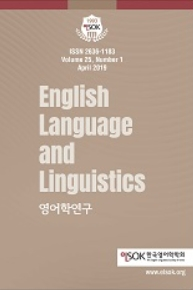상세검색
최근 검색어 전체 삭제
다국어입력
즐겨찾기0KCI등재 학술저널

# Significance Test of N-grams Using Bi-grams and χ 2-test

• 등재여부 : KCI등재
• 2020.04
• 61 - 84 (24 pages)
• 3

N-grams (or lexical bundles) are important linguistic units both in linguistics and in English teaching, but there have been no or few studies which test the significance of the n-grams. This paper proposes an algorithm which can test the significance of the n-grams. The algorithm proceeds as follows. For any n-gram sequence, we first construct an n×n table. Each cell (fij) in the table is filled with the bi-gram frequencies of wiwj. The table goes through a χ2-test, and statistical significance is calculated. In order to check the validity of our algorithm, we apply the algorithm to two corpora. One is the USA component of International Corpus of English (ICE-USA), and the other is the Korean component of the TOEFL11 corpus (TOEFL11-Korean). From two corpora, we extract 3-grams, 4-grams, and 5-grams respectively. Then, we apply the algorithm to each sequence of n-gram and conduct a significance test. We find that 1.0~2.5% of n-grams are statistically significant in the ICE-USA corpus and that 1.4~7.5% are statistically significant in the TOEFL11-Korean corpus. We also observe the tendency that Korean learners tend to overuse a small inventory of n-grams repeatedly.

1. Introduction

2. Previous Studies

3. Research Method

4. Analysis Results

5. Discussion

6. Conclusion